# Answered Essay: C++ PROGRAMMING QUESTION

C++ PROGRAMMING QUESTION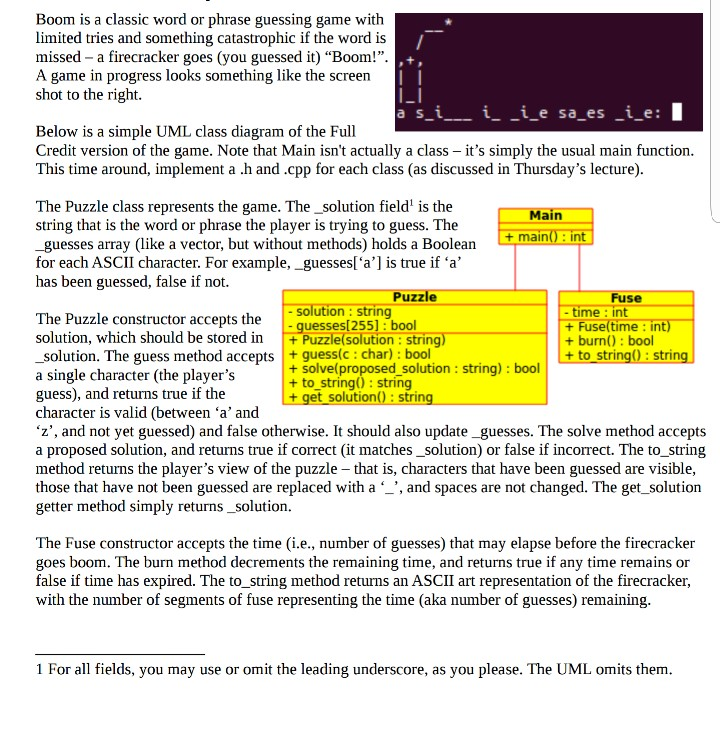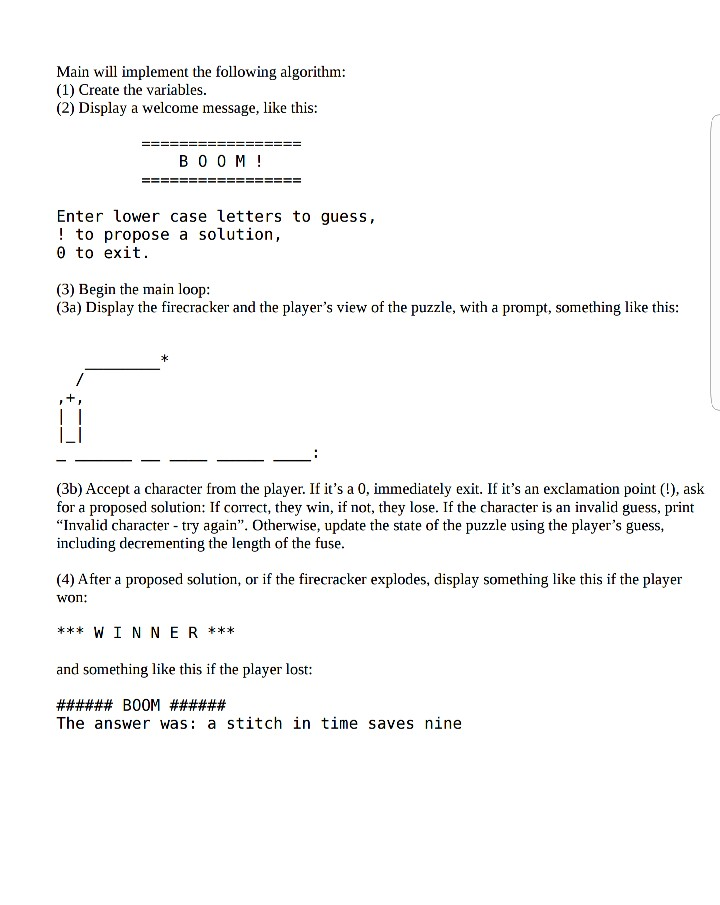Boom is a classic word or phrase guessing game with limited tries and something catastrophic if the word is missed – a firecracker goes (you guessed it) “Boom!”. A game in progress looks something like the screen shot to the right asi i i e sa es i e: I Below is a simple UML class diagram of the Full Credit version of the game. Note that Main isn’t actually a class it’s simply the usual main function This time around, implement a .h and .cpp for each class (as discussed in Thursday’s lecture) The Puzzle class represents the game. The_solution field1 is the string that is the word or phrase the player is trying to guess. The -guesses array (like a vector, but without methods) holds a Boolean for each ASCII character. For example-guesses[a] is true if a has been guessed, false if not. ain + main: int Puzzle Fuse string me: int The Puzzle constructor accepts the solution, which should be stored in Puzzle(solution: string solution. The guess method accepts +guess(c: char): bool a single character (the player’s guess), and returns true if the character is valid (between a and ‘z’, and not yet guessed) and false otherwise. It should also update_guesses. The solve method accepts a proposed solution, and returns true if correct (it matches_solution) or false if incorrect. The to_string method returns the player’s view of the puzzle – that is, characters that have been guessed are visible, those that have not been guessed are replaced with a ‘_’, and spaces are not changed. The get solution getter method simply returns_solution. guesses 255]: bool time int + burn(): bool + to strin strin + solve(proposed solution: string): bool + to string): string + get solution): strin The Fuse constructor accepts the time (i.e., number of guesses) that may elapse before the firecracker goes boom. The burn method decrements the remaining time, and returns true if any time remains or false if time has expired. The to_string method returns an ASCII art representation of the firecracker with the number of segments of fuse representing the time (aka number of guesses) remaining 1 For all fields, you may use or omit the leading underscore, as you please. The UML omits them

#include<iostream>

#include<vector>

#include<array>

#include<algorithm>

#include<map>

#include<set>

#include<hash_map>

#include<hash_set>

#include<list>

#include<queue>

#include<cmath>

#include<stack>

#include<deque>

#include<unordered_map>

#include<unordered_set>

#include<string>

#include<memory>

#include<stdlib.h>

#include<fstream>

#include <iostream>

#include <string>

#include<cassert>

using namespace std;

class Fuse{

private:

int _time;

public:

Fuse(int t) : _time(t){}

bool burn() {

_time = _time – 1;

if (_time <= 0)

return false;

else

return true;

}

string to_string() {

string temp = “”;

int i = 0;

for (i = 0; i < _time; i++)

temp = temp + ‘-‘;

temp = temp + ‘*’;

return temp;

}

};

class Puzzle{

private :

string _solution;

bool _guesses;

public:

Puzzle(string solution) : _solution(solution) {

int i = 0;

for (i = 0; i < 26; i++)

_guesses[i] = false;

}

bool guess(char c) {

//cout << _solution.find(c) << endl;

if (c >= ‘a’ && c <= ‘z’ && _solution.find(c) >= 0 && _solution.find(c)<_solution.length() && _guesses[c – ‘a’] == false) {

_guesses[c-‘a’] = true;

return true;

}

else

return false;

}

bool solve(string proposed_solution) {

if (proposed_solution == _solution)

return true;

else

return false;

}

string to_string() {

string temp = “”;

int i;

for (i = 0; i < _solution.length(); i++) {

if ( _solution[i] >=’a’ && _solution[i]<=’z’ && _guesses[_solution[i]-‘a’])

temp = temp + _solution[i];

else if (_solution[i] >= ‘a’ && _solution[i] <= ‘z’)

temp = temp + ‘_’;

else

temp = temp + _solution[i];

}

temp = temp + “:”;

return temp;

}

string getSolution() {

return _solution;

}

};

void display(string time, string solution) {

cout << ” ” << time<<endl;

cout << ” /” << endl;

cout << “,+,” << endl;

cout << “| |” << endl;

cout << “| |” << endl;

cout << ” – ” << endl;

cout << solution << endl;

}

int main() {

Puzzle puz(“a stitch in time saves nine”);

Fuse fus(5);

bool flag = true;

string proposed_solution;

char ch;

cout << “================” << endl;

cout << ” B O O M ! ” << endl;

cout << “================” << endl<<endl;

cout << “Enter lower case letters to guess,” << endl;

cout << “! to propose a solution” << endl;

cout << “0 to exit” << endl;

display(fus.to_string(), puz.to_string());

while (flag) {

cin >> ch;

if (ch == ‘!’) {

cout << “Enter proposed solution ” << endl;

fflush(stdin);

getline(cin, proposed_solution);

if (puz.solve(proposed_solution)) {

cout << “*** W I N N E R ***” << endl;

flag = false;

}

else {

cout << “###### B O O M ######” << endl;

cout << “The answer was : ” << puz.getSolution() << endl;

flag = false;

}

}

else if (ch == ‘0’) {

flag = false;

}

else {

if (puz.guess(ch)){

bool t = fus.burn();

if (!t) {

cout << “###### B O O M ######” << endl;

cout << “The answer was : ” << puz.getSolution() << endl;

flag = false;

break;

}

display(fus.to_string(), puz.to_string());

}

else{

cout << “Invalid character – try again” << endl;

}

}

}

return 0;

}

Sample ScreenShot of Program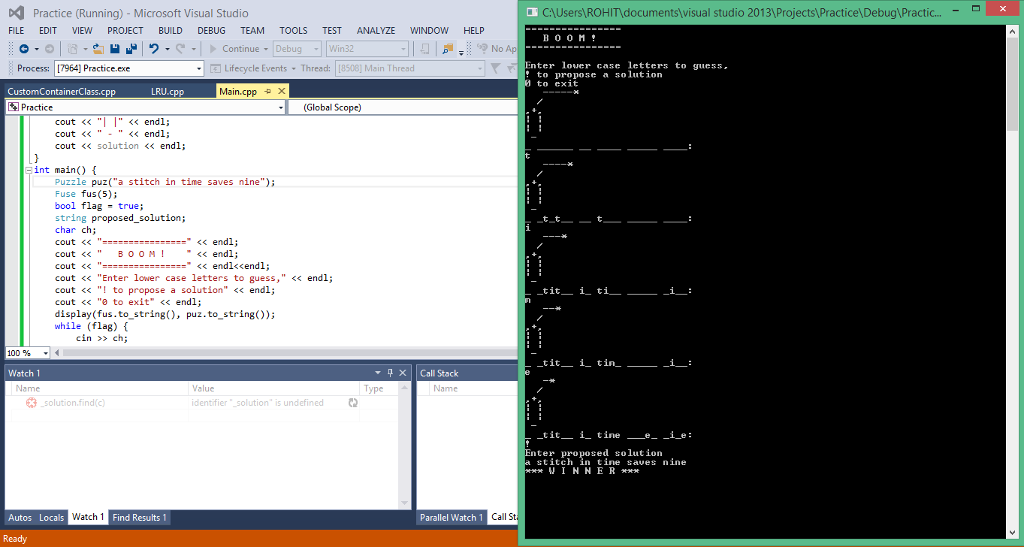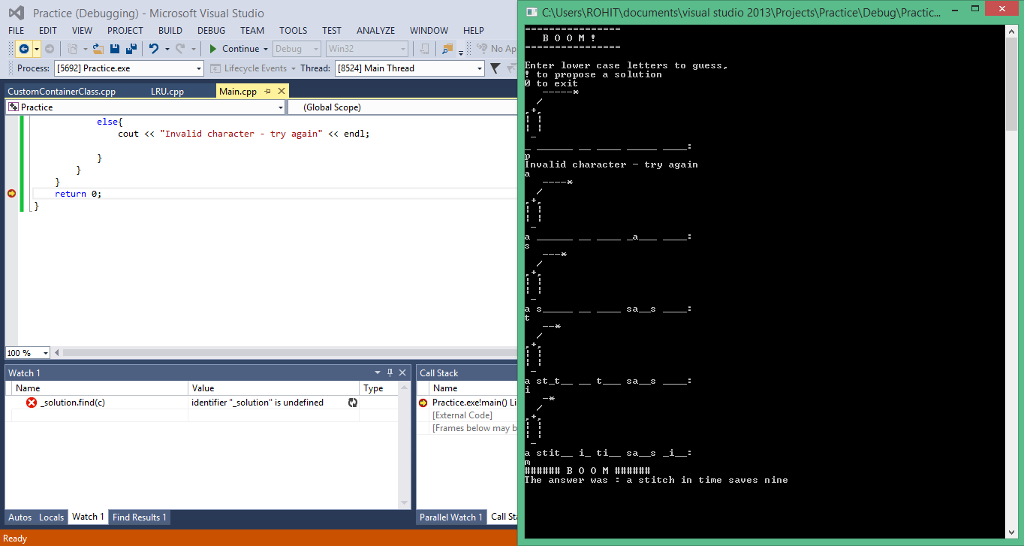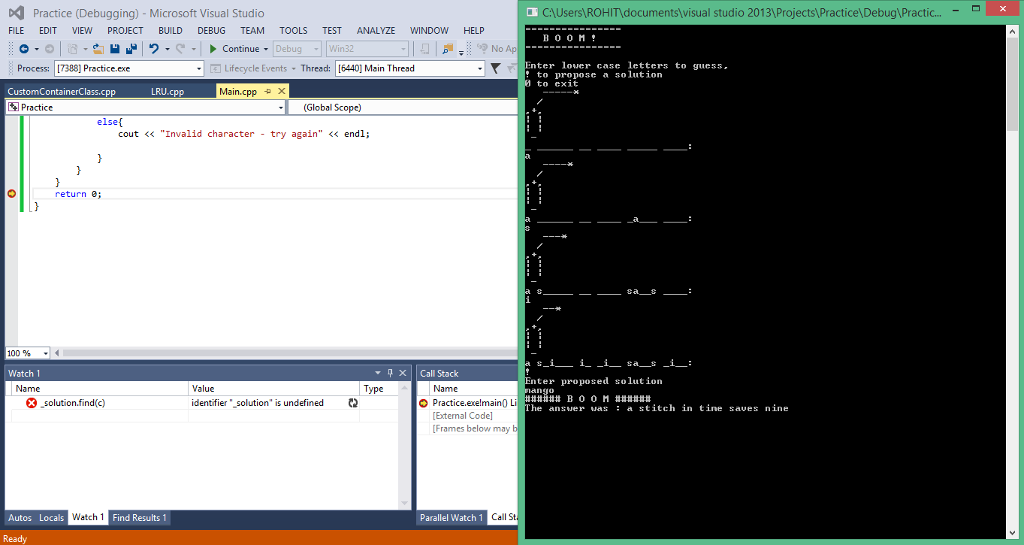Pages (550 words)
Approximate price: -

Help Me Write My Essay - Reasons:Best Online Essay Writing Service

We strive to give our customers the best online essay writing experience. We Make sure essays are submitted on time and all the instructions are followed.Our Writers are Experienced and Professional

Our essay writing service is founded on professional writers who are on stand by to help you any time.Free Revision Fo all Essays

Sometimes you may require our writers to add on a point to make your essay as customised as possible, we will give you unlimited times to do this. And we will do it for free.Timely Essay(s)

We understand the frustrations that comes with late essays and our writers are extra careful to not violate this term. Our support team is always engauging our writers to help you have your essay ahead of time.Customised Essays &100% Confidential

Our Online writing Service has zero torelance for plagiarised papers. We have plagiarism checking tool that generate plagiarism reports just to make sure you are satisfied.Try it now!

## Calculate the price of your order

Total price:
\$0.00

How it works?Fill in the order form and provide all details of your assignment.Proceed with the payment

Choose the payment system that suits you most.HOW OUR ONLINE ESSAY WRITING SERVICE WORKS

Let us write that nagging essay.By clicking on the "PLACE ORDER" button, tell us your requires. Be precise for an accurate customised essay. You may also upload any reading materials where applicable.Pick A & Writer

Our ordering form will provide you with a list of writers and their feedbacks. At step 2, its time select a writer. Our online agents are on stand by to help you just in case.Editing (OUR PART)

At this stage, our editor will go through your essay and make sure your writer did meet all the instructions.